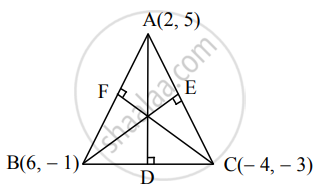Advertisement Remove all ads

# Find equations of altitudes of the triangle whose vertices are A(2,5), B(6,–1) and C(–4,–3). - Mathematics and Statistics

Sum

Find equations of altitudes of the triangle whose vertices are A(2,5), B(6,–1) and C(–4,–3).

Advertisement Remove all ads

#### SolutionLet AD be the altitude through A.

∴ AD is perpendicular to BC and

slope of BC = (-3 -( - 1))/(-4 - 6)

= (-3 + 1)/(-10)

= 1/5

∴ slope of AD = – 5

Since altitude AD passes through the point A (2, 5) and has slope - 5, equation of the altitude AD is

y – 5 = –5(x – 2)

∴ y – 5 = –5x + 10

∴ 5x + y – 15 = 0

Let BE be the altitude through B.

∴ BE is perpendicular to AC and

slope of AC = (-3 - 5)/(-4 - 2)

= (-8)/(-6)

= 4/3

∴ slope of BE = -3/4

Since altitude BE passes through the point B (6, – 1) and has slope -3/4, equation of the altitude BE is

y – (– 1) = -3/4(x - 6)

∴ 4y + 4 = – 3x + 18

∴ 3x + 4y – 14 = 0

Let CF be the altitude through C.

∴ CF is perpendicular to AB and

slope of AB = (5 - ( - 1))/(2 - 6)

= 6/(-4)

= -3/2

∴ slope of CF = 2/3

Since altitude CF passes through C(– 4, – 3) and has slope 2/3, the equation of altitude CF is

y – (– 3) = 2/3[x - (- 4)]

∴ 3y + 9 = 2x + 8

∴ 2x – 3y – 1 = 0

Hence, equations of the altitudes of the ΔABC are

5x + y – 15 = 0, 3x + 4y – 14 = 0 and 2x – 3y – 1 = 0.

Is there an error in this question or solution?
Advertisement Remove all ads

#### APPEARS IN

Advertisement Remove all ads
Advertisement Remove all ads
Share
Notifications

View all notifications

Forgot password?